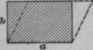## Lines And Plane Surfaces## Triangle

Right Triangle.Oblique Triangle.

If altitude or height h and base 6 are known:

Area = 1/2 b h.

If the three sides are known:

Let s = 1/2(a + b + c).* This formula, while given as an exercise in formulas, is also useful in calculating directly the size of a square wooden column, instead of ascertaining it by trial, as is usual.Area = ab.

## Trapezoid

Area = 1/2h(a + b).## Trapezium

Divide into two triangles and a trapezoid.

Area =or, area## Regular Polygons

Divide the polygon into equal triangles and find the sum of the partial areas. Otherwise, square the length of one side and multiply by proper number from the following table:

 Name. No. Sides. Multiplier. Triangle 3 .433 Square 4 1.000 Pentagon 5 1.720 Hexagon 6 2.598 Heptagon 7 3.634 Octagon 8 4.828 Nonagon 9 6.182 Decagon 10 7.694## Irregular Areas

Divide the area into trapezoids, triangles, parts of circles, etc., and find the sum of the partial areas.

If the figure is very irregular, the approximate area may be found as follows: Divide the figure into trapezoids by equidistant parallel lines b,c,d, etc. The lengths of these lines being measured, then, calling a the first and n the last length, and y the width of strips,

Area = y{(a+n)/2+b+c+etc+m}